﻿ 试位法的一种改进及其收敛性分析 An Improvement of the Regular Falsi Method and Its Convergence Analysis

Vol. 08  No. 08 ( 2019 ), Article ID: 31800 , 7 pages
10.12677/AAM.2019.88167

An Improvement of the Regular Falsi Method and Its Convergence Analysis

Bo Yu, Junqing Jia

School of Mathematics and Statistics, Wuhan University, Wuhan Hubei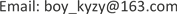Received: Aug. 1st, 2019; accepted: Aug. 15th, 2019; published: Aug. 22nd, 2019ABSTRACT

The Regular Falsi method is an algorithm for finding the root of a univariate nonlinear equation by numerical method. The method is simple and easy. The Regular Falsi method is an improvement of the Bisection method and is superior to the Bisection method in most cases. In this paper, an improved algorithm of the test method is given by combining the dichotomy and the test position method. The convergence of the algorithm is proved and the convergence index is estimated.

Keywords:Regular Falsi Method, Illinois Method, Numerical Solution, Nonlinear Equations1. 引言

$c=b-\frac{f\left(b\right)\left(b-a\right)}{f\left(b\right)-f\left(a\right)}$

$c=b-\frac{f\left(b\right)\left(b-a\right)}{f\left(b\right)-\gamma f\left(a\right)}$

2. 对参数的理解

2.1. 二分法

2.2. 试位法

$f\left(x\right)$ 在闭区间 $\left[a,b\right]$ 上连续且在端点处异号。以连接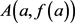$B\left(b,f\left(b\right)\right)$ 的直线段L近似代替函数 $f\left(x\right)$ 的图像，而以L与x轴的交点坐标c作为 $f\left(x\right)$ 零点的近似。

$\frac{0-f\left(b\right)}{c-b}=\frac{f\left(b\right)-f\left(a\right)}{b-a}$

$c=b-\frac{f\left(b\right)\left(b-a\right)}{f\left(b\right)-f\left(a\right)}$

$f\left(c\right)\ne 0$，则方程的实际根落在 $\left[a,c\right]$$\left[c,b\right]$ 中，选择这个区间重置原区间。重复如上过程直至 $|f\left(c\right)|$ 前后两次迭代结果的差小于事先设定的误差。

3. 收敛性分析

3.1. 收敛性

$f\left(x\right)\in C\left[a,b\right]$，对于迭代产生的序列 $\left[{a}_{n},{b}_{n}\right]$，根据试位法的迭代原则知序列 $\left\{{a}_{n}\right\}$ 单调不减，序列 $\left\{{b}_{n}\right\}$ 单调不增，因此收敛，分别设其极限为 ${d}_{1}$${d}_{2}$，且有 ${d}_{1}\le {d}_{2}$

1) 假设 ${d}_{1}$${d}_{2}$ 都不是 $f\left(x\right)$ 的根。

${c}_{n}-{a}_{n}=\frac{f\left({a}_{n}\right)\left({a}_{n}-{b}_{n}\right)}{f\left({b}_{n}\right)-f\left({a}_{n}\right)}$${c}_{n}-{b}_{n}=\frac{f\left({b}_{n}\right)\left({a}_{n}-{b}_{n}\right)}{f\left({b}_{n}\right)-f\left(an\right)}$

$|f\left(x\right)|$$\left[a,b\right]$ 上有界，设为M。则对于任意的正整数n，都成立

$|{a}_{n+1}-{b}_{n+1}|\le \mathrm{max}\left(|{c}_{n}-{a}_{n}|,|{c}_{n}-{b}_{n}|\right)\le \frac{M|{a}_{n}-{b}_{n}|}{M+\epsilon }$

$|{a}_{N+k}-{b}_{N+k}|\le {\left(\frac{M}{M+\epsilon }\right)}^{k}|{a}_{N}-{b}_{N}|$

$\underset{n\to +\infty }{\mathrm{lim}}{a}_{n}=\underset{n\to +\infty }{\mathrm{lim}}{b}_{n}={d}_{1}$

2) 假设 ${d}_{1}$ 是方程的根， ${d}_{2}$ 不是方程的根。

$|{c}_{n}-{a}_{n}|\le \frac{|f\left({a}_{n}\right)||{a}_{n}-{b}_{n}|}{|f\left({b}_{n}\right)|}$

$\underset{n\to +\infty }{\mathrm{lim}}{c}_{n}={d}_{1}$

3) 假设 ${d}_{1}$${d}_{2}$ 都是方程的根。若 ${d}_{1}\ne {d}_{2}$，则存在一子列 ${c}_{i}$ 收敛到 ${d}_{1}$，或有另一子列收敛到 ${d}_{2}$，即 $\underset{n\to +\infty }{\mathrm{lim}}{c}_{n}={d}_{1}$

${d}_{1}-{a}_{n}<\delta$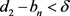$\frac{f\left({b}_{n}\right){a}_{n}-{b}_{n}f\left({a}_{n}\right)}{f\left({b}_{n}\right)-f\left({a}_{n}\right)}<{d}_{1}$

$f\left({b}_{n}\right)\le \frac{{b}_{n}-{d}_{1}}{{d}_{1}-{a}_{n}}$。不妨设 $f\left({a}_{n}\right)<0$$f\left({b}_{n}\right)>0$。为了序列 $\left\{{b}_{n}\right\}$ 收敛到 ${d}_{2}$ ( ${b}_{n}>{d}_{2}$ )，必须有对于比n大的m，满足 ${c}_{m}>{d}_{2}$，这表明：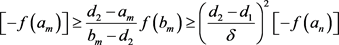$\left[-f\left({a}_{p}\right)\right]\ge \frac{{d}_{2}-{a}_{m}}{{b}_{m}-{d}_{2}}f\left({b}_{m}\right)\ge {\left(\frac{{d}_{2}-{d}_{1}}{\delta }\right)}^{2}\left[-f\left({a}_{n}\right)\right]$

3.2. 收敛速度

${c}_{n+1}-{x}^{*}=\frac{\left({b}_{n}-{x}^{*}\right)f\left({a}_{n}\right)-f\left({b}_{n}\right)\left({a}_{n}-{x}^{*}\right)}{f\left({a}_{n}\right)-f\left(bn\right)}$

$\frac{\text{d}}{\text{d}x}\left[\frac{f\left(x\right)}{x-{x}^{*}}\right]=\frac{{f}^{\prime }\left(x\right)\left(x-{x}^{*}-f\left(x\right)\right)}{{\left(x-{x}^{*}\right)}^{2}}$

$\frac{{c}_{n+1}-{x}^{*}}{\left({b}_{n+1}-{x}^{*}\right)\left({a}_{n+1}-{x}^{*}\right)}=\frac{{f}^{\prime }\left(\xi \right)\left(\xi -{x}^{*}\right)-{f}^{\prime }\left(\xi \right)}{{f}^{\prime }\left(\xi \right){\left(x-{x}^{*}\right)}^{2}}$

$0=f\left({x}^{*}\right)=f\left(\xi \right)+{f}^{\prime }\left(\xi \right)\left({x}^{*}-\xi \right)+\frac{{f}^{″}\left(\theta \right)}{{\left({x}^{*}-\xi \right)}^{2}}$

${c}_{n+1}-{x}^{*}=\frac{{f}^{″}\left(\theta \right)}{2f\left(\xi \right)}\left({b}_{n}-{x}^{*}\right)\left({a}_{n}-{x}^{*}\right)$

${\epsilon }_{n}={c}_{n}-{x}^{*}$${{\eta }^{}}_{n}={b}_{n}-{x}^{*}$${{\eta }^{\prime }}_{n}={a}_{n}-{x}^{*}$，则上式可写为

${\epsilon }_{n+1}=\frac{{f}^{″}\left(\theta \right)}{2f\left(\xi \right)}{\eta }_{n}{{\eta }^{\prime }}_{n}$

$|{\epsilon }_{n+1}|\le \frac{M}{2m}|{\eta }_{n}||{{\eta }^{\prime }}_{n}|$

$K=\frac{M}{2m}$ 乘不等式的两边，有

$K|{\epsilon }_{n+1}|\le {K}^{2}|{\eta }_{n}||{{\eta }^{\prime }}_{n}|$

$d=\mathrm{max}\left\{{d}_{0},{{d}^{\prime }}_{0}\right\}\le 1$

4. Illinois算法

4.1. 收敛性

Illinois算法很好地避免了不动点的出现，近似根从两端收敛到精确根，即迭代生成的新区间的长度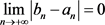。基于这一条件，根据区间套定理以及一致连续定理，我们给出一下证明。

$|{b}_{n}-{a}_{n}|=|{b}_{n}-{x}^{*}|+|{x}^{*}-{a}_{n}|<\delta$

$|f\left({b}_{n}\right)-f\left({a}_{n}\right)|<\epsilon$，即

$|f\left({b}_{n}\right)-0|+|0-f\left({a}_{n}\right)|<\epsilon$

$\underset{n\to +\infty }{\mathrm{lim}}{b}_{n}={x}^{*}=\underset{n\to +\infty }{\mathrm{lim}}{a}_{n}$

$\underset{n\to +\infty }{\mathrm{lim}}f\left({b}_{n}\right)=f\left({x}^{*}\right)=\underset{n\to +\infty }{\mathrm{lim}}f\left(an\right)$

$\underset{n\to +\infty }{\mathrm{lim}}{c}_{n}={x}^{*}$

$\underset{n\to +\infty }{\mathrm{lim}}f\left({c}_{n}\right)=f\left({x}^{*}\right)=0$

4.2. 收敛速度

$\beta =\frac{{C}_{2}}{{C}_{1}}$

$L={\beta }^{2}-\frac{{C}_{3}}{{C}_{1}}$

${\epsilon }_{i+1}\sim L{\epsilon }_{i}{\epsilon }_{i-1}$

Illinois算法一次迭代包括先进行一次试位法，在进行两次修正  ，则

${\epsilon }_{i+3}\sim {L}^{2}{\left({\epsilon }_{i}\right)}^{3}$

5. 函数实例

5.1. 不动点的情况

5.2. 算例对比测试Table 1. Comparison of examples between regular falsi method and illinois method

6. 总结

An Improvement of the Regular Falsi Method and Its Convergence Analysis[J]. 应用数学进展, 2019, 08(08): 1432-1438. https://doi.org/10.12677/AAM.2019.88167

1. 1. Dowell, M. and Jarratt, P. (1971) A Modified Regular Falsi Method for Computing the Root of an Equation. BIT Nu-merical Mathematics, 11, 168-174. https://doi.org/10.1007/BF01934364

2. 2. Anderson, N. and Björck, Å. (1973) A New High Order Method of Regula Falsi Type for Computing a Root of an Equation. BIT Numerical Mathematics, 13, 253-264. https://doi.org/10.1007/BF01951936

3. 3. Young, D. and Gregory, R. (1972) A Survey of Numerical Mathmatics. Addison-Wesley, Reading.

4. 4. Ford, J. (1995) Improved Algorithms of Illinois-Type for the Numerical Solution of Nonlinear Equations. ACM Transactions on Mathematical Software, 30, 64-85.

5. 5. Szidarovszky, F. and Yakowitz, S. (1980) Principles and Procedures of Numerical Analysis, American Scientist. Plenum Press, New York.